# Search by Topic

Filter by: Content type:
Age range:
Challenge level:

### There are 25 results

Broad Topics > Vectors and Matrices > Vectors### Vector Racer

##### Age 11 to 16 Challenge Level:

The classic vector racing game.### Vector Journeys

##### Age 14 to 18 Challenge Level:

Charlie likes to go for walks around a square park, while Alison likes to cut across diagonally. Can you find relationships between the vectors they walk along?### Vector Walk

##### Age 14 to 18 Challenge Level:

Starting with two basic vector steps, which destinations can you reach on a vector walk?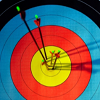### Spotting the Loophole

##### Age 14 to 16 Challenge Level:

A visualisation problem in which you search for vectors which sum to zero from a jumble of arrows. Will your eyes be quicker than algebra?### Areas of Parallelograms

##### Age 14 to 16 Challenge Level:

Can you find the area of a parallelogram defined by two vectors?### Cubestick

##### Age 16 to 18 Challenge Level:

Stick some cubes together to make a cuboid. Find two of the angles by as many different methods as you can devise.### Square Coordinates

##### Age 11 to 14 Challenge Level:

A tilted square is a square with no horizontal sides. Can you devise a general instruction for the construction of a square when you are given just one of its sides?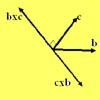### Multiplication of Vectors

##### Age 16 to 18

An account of multiplication of vectors, both scalar products and vector products.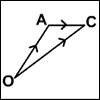### An Introduction to Vectors

##### Age 14 to 18

The article provides a summary of the elementary ideas about vectors usually met in school mathematics, describes what vectors are and how to add, subtract and multiply them by scalars and indicates. . . .### Which Twin Is Older?

##### Age 16 to 18

A simplified account of special relativity and the twins paradox.### 8 Methods for Three by One

##### Age 14 to 18 Challenge Level:

This problem in geometry has been solved in no less than EIGHT ways by a pair of students. How would you solve it? How many of their solutions can you follow? How are they the same or different?. . . .##### Age 16 to 18 Challenge Level:

A quadrilateral changes shape with the edge lengths constant. Show the scalar product of the diagonals is constant. If the diagonals are perpendicular in one position are they always perpendicular?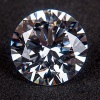### Vector Gem Collector

##### Age 14 to 18 Challenge Level:

Use vectors to collect as many gems as you can and bring them safely home!### Another Triangle in a Triangle

##### Age 16 to 18 Challenge Level:

Can you work out the fraction of the original triangle that is covered by the green triangle?### From Point to Point

##### Age 14 to 16 Short Challenge Level:

Can you combine vectors to get from one point to another?### Nine Eigen

##### Age 16 to 18 Challenge Level:

Explore how matrices can fix vectors and vector directions.### Matrix Meaning

##### Age 16 to 18 Challenge Level:

Explore the meaning behind the algebra and geometry of matrices with these 10 individual problems.### Polygon Walk

##### Age 16 to 18 Challenge Level:

Go on a vector walk and determine which points on the walk are closest to the origin.### Fix Me or Crush Me

##### Age 16 to 18 Challenge Level:

Can you make matrices which will fix one lucky vector and crush another to zero?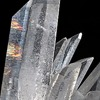### Coordinated Crystals

##### Age 16 to 18 Challenge Level:

Explore the lattice and vector structure of this crystal.### V-P Cycles

##### Age 16 to 18 Challenge Level:

Form a sequence of vectors by multiplying each vector (using vector products) by a constant vector to get the next one in the seuence(like a GP). What happens?### Air Routes

##### Age 16 to 18 Challenge Level:

Find the distance of the shortest air route at an altitude of 6000 metres between London and Cape Town given the latitudes and longitudes. A simple application of scalar products of vectors.##### Age 16 to 18 Challenge Level:

As a quadrilateral Q is deformed (keeping the edge lengths constnt) the diagonals and the angle X between them change. Prove that the area of Q is proportional to tanX.### The Use of Mathematics in Computer Games

##### Age 16 to 18

An account of how mathematics is used in computer games including geometry, vectors, transformations, 3D graphics, graph theory and simulations.### A Knight's Journey

##### Age 14 to 18

This article looks at knight's moves on a chess board and introduces you to the idea of vectors and vector addition.